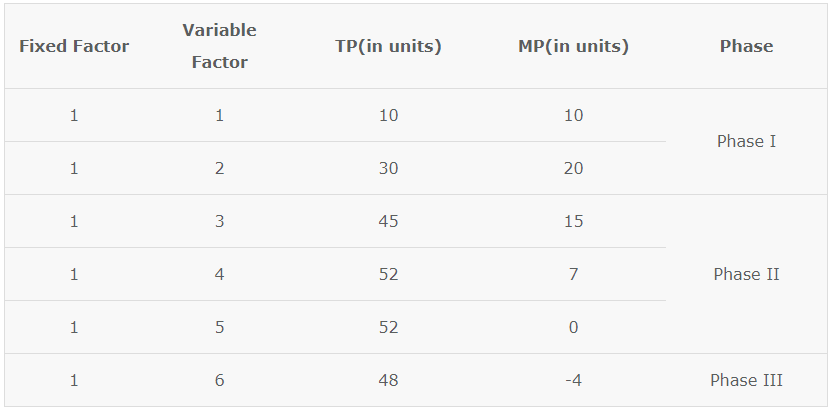# Law of Variable Proportion

In the short run, the output is affected due to change in variable input, assuming no change in other factors. As there is change in variable input only, the ratio between different inputs tends to change at different levels of output. This relationship is explained by Law of Variable proportions. Before we move on to the law of variable proportions, let us take a look at what is meant by returns to a factor.

## Law of Variable Proportions

### Returns to a Factor

Returns to a factor refers to the resultant increase in the total product (return) when only one factor is increased, keeping all other factors fixed. In the short run, when one input is variable and all other inputs are fixed, the firm’s production function exhibits the law of variable proportions. The law of variable proportions is one of the most important laws of production. It shows the nature of the rate of change in output due to a change in only one variable factor of production.

### Statement of Law of variable Proportions

Law of Variable Proportions states that as we increase quantity of only one input keeping other inputs fixed, total product (TP) initially increases at an increasing rate, then at a decreasing rate and finally at a negative rate.

According to the Law of Variable Proportions, the changes and behaviour of TP and MP can be classified into three phases. Let us understand each phase with the help of a diagram.#### Phase I – Increasing Returns to Factor

In the first phase every additional unit of variable factor adds more and more to the total product. TP increases at an increasing rate and MP of each variable factor rises. As seen in the diagram, TP increases at an increasing rate till point A and MP rises till it reaches point B. This is because, in this phase the fixed factors are too large and the variable factors are few. Therefore adding more and more units of variable factors leads to better utilisation of the fixed factors and thus TP increases at an increasing rate.

#### Phase II – Diminishing Returns to a Factor

In the next phase, every additional unit of variable factor contributes lesser and lesser to the total output. TP increases at a diminishing rate and MP of each variable factor decreases. In the diagram, TP rises till it is maximum at point M and MP decreases till it is zero at point S. When TP is maximum, MP is zero. Diminishing returns occur in this phase because, the optimum use of fixed factor has already been made after which the marginal return of variable factors start to diminish. Another contributing reason is that fixed factors and variable factors are imperfect substitutes for one another.

#### Phase III – Negative Returns to a Factor

In the third phase, the additional units of variable factor cause the TP to decline and MP becomes negative. Thus, this phase is known as negative returns to a factor. The third phase starts after point M on the TP curve and point S on the MP curve. In this phase MP of each variable factor is negative. This is because of the limitation of fixed factors, poor coordination between variable and fixed factors and decrease in efficiency of variable factor.

### Assumptions of Law of Variable Proportions

1. It operates in the short run, as factors are classified as variable and fixed.
2. The law applies to all fixed factors including land.
3. Different units of different variable factors can be combined with fixed factor.
4. This law applies to the field of production only.
5. The effect of change in output due to change in variable factor can be easily pin-pointed.
6. Factors of production are imperfect substitutes of each other beyond a certain limit.
7. The level of technology is constant.
8. All variable factors are assumed to be equally efficient.

### Phase Operation

A rational producer will always prefer to operate in the second phase of the law of variable proportions. In Phase I every additional unit of variable factor gives increasing amount of output. This means that more profits can be made and the producer increases production with more units of variable factor.

In the third phase of the law of variable proportions, marginal product of additional units of variable factors is negative and the total product is declining. This means that the producer will lose profits. Therefore, no rational consumer will choose to operate in this phase. This concludes that a produce will aim to operate in the second phase as TP is maximum and MP is positive.